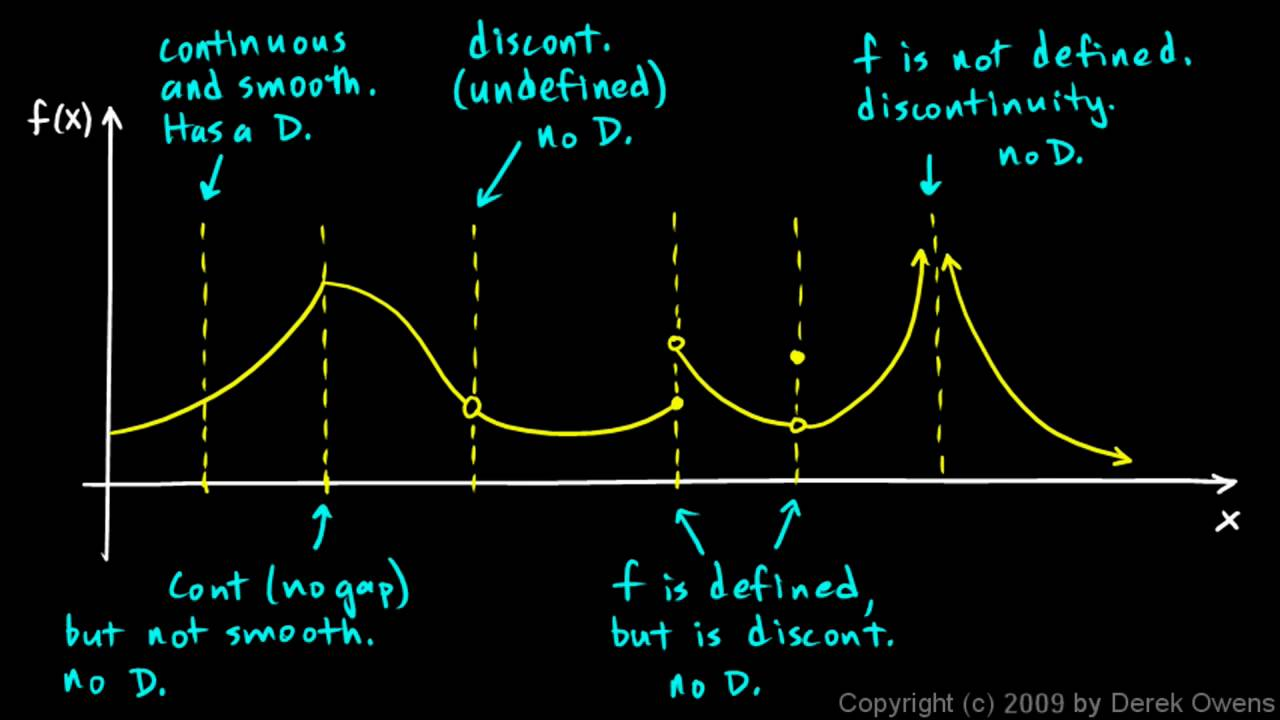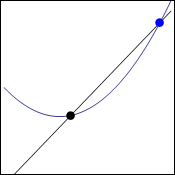# Derivatives made easy 2) Non-differentiable functions

Hi,
so I continue with this tutorial. Today I will cover when function is not differentiable. If you want to read the basics check out the last post, it links to limits and continuity which will be important today.

Just to remind ourselves here is the formula that I explained last time:Basics

It uses limit so logically if the point we are trying to derive is not continous, which means that the limit does not exist there we can not derive. This is not the only thing that can happen to us. Sometimes function is completely continous but from negative side it grows exponentially and from positive side it is linear. Check out the nice picture below:Continuity

From the whole picture the most important point is the second one. It says: “continous (no gap) but not smooth, not differentiable”. To find this out algebraically you need to know the equations of both left and right part of the function.

If you know them you will insert it into the derivation equation that we used last time as f(x). You have to add h of course as it is the difference between your two points. If both of your solutions equal (right and left side) you know that the function IS differentiable.

Dragallur

PS: ask me if you want to expand any part of the post. I have no problem whatsoever with it and it will make me even happy to write some extra parts.

Yes function can be made up of different parts only on intervals. You can even write something like (odd Xs are equal to 2).

# Derivatives made easy 1) Slope on curve

Hi,
today I am going to explain one fundamental equation that is used to calculate derivatives.

Derivative of a function basically says what is the rate of change or the slope of that function. You can have both rate of change of whole function which gives you another function or just at one point which equals to some number. The fundamental thing is to find the slope. Last time I explained this only for a line, not curve. On line the slope is constant but not on curve.Tangent and secant line.

On the gif above you can see a function. There is a black point that is on the same place. Blue point is getting closer and closer with each step. All the time the black line creates secant line to the curve, it cuts it on two points. When it is red it is tangent line which means that it does not cuts it at all. It is the only one step in which it is just touching. To get the rate of change of curve you need to find this tangent line. Lets call the distance between the two points “h”. The tangent line will exist when h approaches zero.THE equation. Sometimes f'(x) is written on the left.

This thing above is the equation that we will get when we take coordinates of both points.

black point(  x;f(x)   )                    
blue point  (  x+h;f(x+h)  )           

Just to clear things out, the first thing in the brackets is the x coordinate and the second y coordinate. As I said blue point is moved to side by the distance h and as the distance approaches 0 we are getting tangent line of curve. In the last post I showed that you need to only divide the difference of y coordinates by difference of x coordinates. Check it out if you do not know what I mean.

We will do exactly it to get right part of the equation above without the limit. Only h will be left in the denominator because there is the difference in x coordinates which means:

x+h-x=h

Now to get the tangent line we only add the limit and we have got the fundamental equation of derivates. This we can use for any curve or line that we want to derive. The left part is just a notation for derivation.

Dragallur

PS: be sure to check out this page that lets you interactively create secant and tangent lines.

Just in case you do not understand why there is f(), it is basicly the same as y though we are in functions so we use this kind of notation and yes, f(x)=y. We just add h to get the y coordinate of blue point.